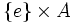# Complements to normal subgroup need not be automorphic

(diff) ← Older revision | Latest revision (diff) | Newer revision → (diff)

## Statement

Suppose$G$ is a group,$N$ is a normal subgroup, and$H$ and$K$ are permutable complements to$N$ in$G$. Then, it is not necessary that there exists an automorphism of$G$ sending$H$ to$K$.

## Proof

### A generic example

Let$A$ be any non-Abelian group. Consider$G = A \times A$ and the subgroup$N = A \times \{ e \}$. Let$H$ be the subgroup$\{ e \} \times A$ and$K$ be the subgroup$\{ (a,a) \mid a \in A \}$.

Note that:

•$N$ is normal in$G$: In fact, it is a direct factor of$G$.
•$H$ is a permutable complement to$N$ in$G$.
•$K$ is a permutable complement to$N$ in$G$.
•$H$ is normal in$G$: In fact, it is a direct factor of$G$.
•$K$ is not normal in$G$: Pick$a,b \in A$ such that$a,b$ do not commute. Then, we have$(b,e)(a,a)(b,e)^{-1}) = (bab^{-1},a)$. Thus, a conjugate of an element in$K$ lies outside$K$.